A ferris wheel 80 meters in diameter is boarded at its lowest point (6 O'Clock) from a platform that is 10 meters above ground. The wheel makes one full rotation every 20 minutes, and at time $t = 0$ you are at the loading platform (6 O'Clock). Let $h = f(t)$ denote your height above ground in meters after $t$ minutes.

(a) What is the period of the function $h = f(t)$?
(b) What is the midline of the function $h = f(t)$?
$h =$ Include units in your answer.
(c) What is the amplitude of the function $h = f(t)$?
(d) Consider the six possible graphs of $h = f(t)$ below. Be sure to enlarge each graph and carefully read the labels on the axes in order distinguish the key features of each graph.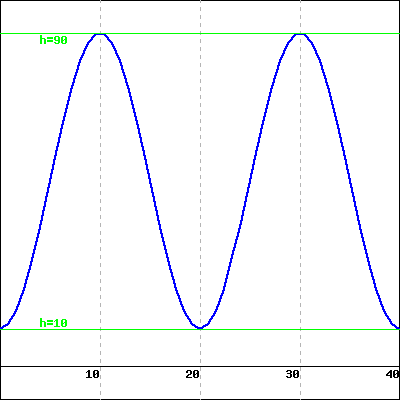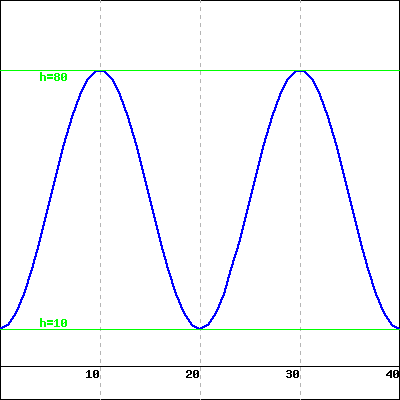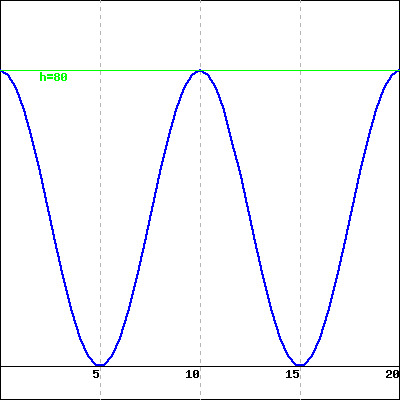A B C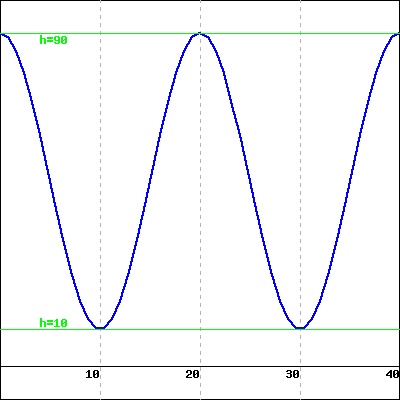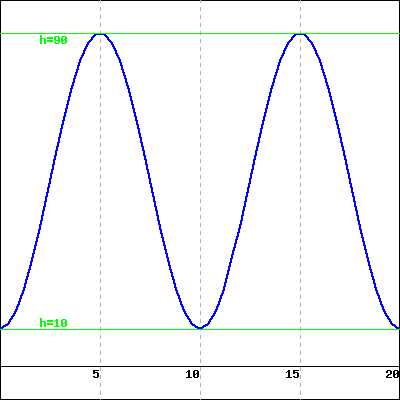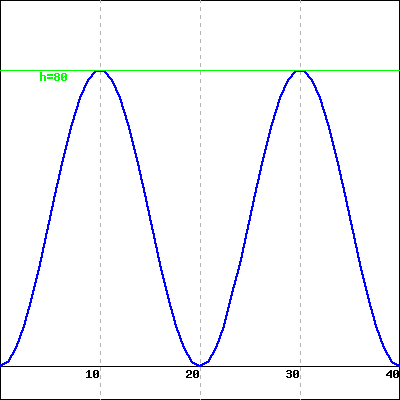D E F